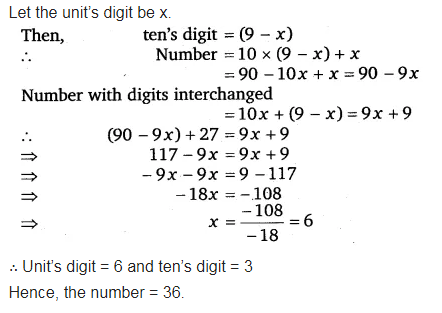# Sum of the digits of a two-digit number is 9. When we interchange the digits

Sum of the digits of a two-digit number is 9. When we interchange the digits, it is found that the resulting new number is greater than the original number by 27. What is the two-digit number ?